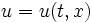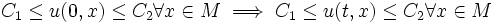Bound-narrowing trajectory

This article defines a property that can be evaluated for a trajectory on the space of functions on a manifold

Definition

Let$M$ be a manifold and$u = u(t,x)$ be a function$\R \times M \to \R$, where:

•$t$ denotes the time parameter, and varies in$\R$
•$x$ denotes the spatial parameter, and varies in$M$

In other words,$u$ is a trajectory (or path) in the space of all functions from$M$ to$\R$.

Then,$u$ is said to be bound-narrowing if it is both max-decreasing and min-increasing or equivalently if it satisfies the following condition:$C_1 \le u(0,x) \le C_2 \forall x \in M \implies C_1 \le u(t,x) \le C_2 \forall x \in M$